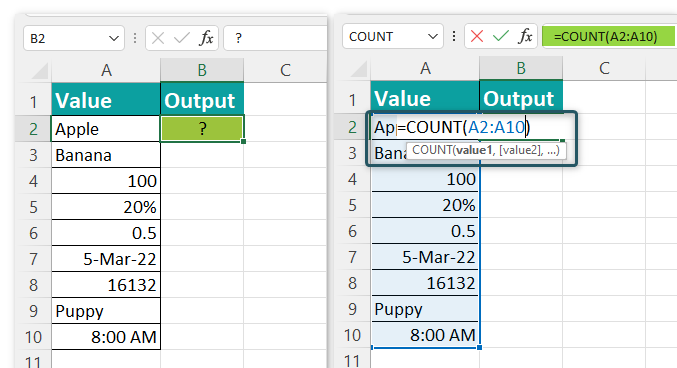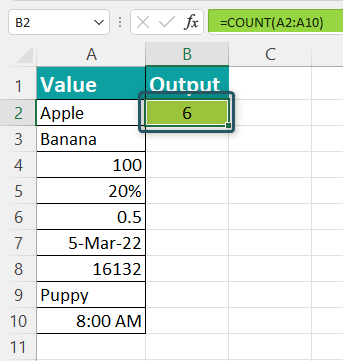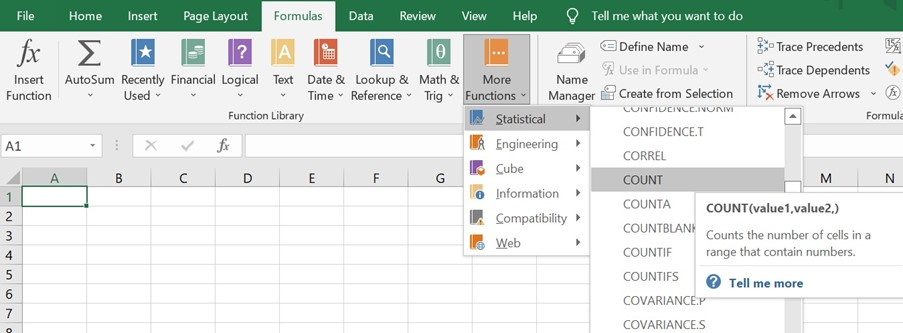# COUNT Excel FunctionArticle byExcelMojo Team## What Is COUNT() Function In Excel?

The COUNT Excel function counts the number of cells or arguments in an array that contains numeric values. It is a mathematical Excel function that returns a numerical value.

For example, the following image shows values that contain numbers and alphabets in column A. We count the cells having numeric values using the COUNT formula in cell B2.

=COUNT(A2:A6)

The output comes as ‘3’.

###### Key Takeaways
• Using the COUNT formula, users can count the cells, cell references, ranges (or all three combined) containing numeric values in Excel.
• At least one argument in the formula is mandatory, while others can be optional. It can handle a maximum of 255 characters and returns the output as 0.
• The function does not work on a non-numeric table array. It ignores text values, errors, logical values, and blank cells.
• It performs simple calculations but combining it with other functions gives amazing results.

### Syntax Of The COUNT() Excel Formula

The image below is an explanation of the arguments accepted by the COUNT Excel function:

• value1 = The first cell reference or the range we want to count. This is the mandatory argument.
• value2 = The second cell reference or the range we want to count. This is the optional argument.

### How To Use COUNT Function?

We can execute the COUNT Excel function in Excel in two ways:

1. Choose the empty cell that will hold the result.
2. Go to the “Formulas” tab and click it.
3. Select “More Functions” from the drop-down menu.
4. Select “Statistical” from the drop-down menu.
5. Select “COUNT” from the drop-down menu.
6. A window called “Function Arguments” appears.
7. As the number of arguments, enter the value in “Value 1” and “Value 2.”
8. Select OK.

#### 2. Manually On The Sheet

• Select the empty cell
• Type =COUNT(
• Double click on the COUNT command
• Select the range of cells or array
• Press “Enter

The image below depicts a set of values in various formats. First, we will try to count the cells having numeric values using the COUNT formula in cell B2.

In the table, the data is reflected as below:

• Column A shows values
• Cell B2 calculates the number of numeric values

The steps to add the given numbers are as follows:

Step 1:  First, we will choose the cell where we want the result to show up. Cell B2 would be the cell in this case.

Step 2:  Next, we will enter the COUNT formula in cell B2.

Step 3: Select the array which ranges from the starting cell address to the ending cell address of the table, i.e., “A2:A7”.

=COUNT(A2:A7)

Step 4: After entering each value in the preceding step, press the “Enter” key. The formula gives the result in cell B2 as ‘2’.

Therefore, the COUNT Excel function counts only the numerical values, not the numbers with a variable.

### Examples

Let us look at some COUNT Excel function examples to understand better how to use it:

#### Example #1 – COUNTA Function In Excel

The COUNTA function is predefined and the family member of the COUNT Excel function. It counts all the cells in the range that has values, both numerical and alphabetical.

The formula of the COUNTA function is as follows:

=COUNTA(value1,[value2],…)

The image below depicts the data of people working in a firm from different locations. The values include their ID, Date of joining, Location, Age, and Gender. Here, we will try to count the number of all cells having numbers and letters using the COUNT Excel function.

In the table, the data is reflected as below:

• Column A shows ID
• Column B contains the Date
• Column C contains the Location
• Column D contains the Age
• Column E contains Gender

The steps to count the number of cells are as follows:

Step 1:  First, we will choose the cell where we want the result to show up. Cell E7 would be the cell in this case.

Step 2:   Enter the formula that needs to count values from the table. Select the value1, which is the range from the starting cell address to the ending cell address of the table, i.e., cell references, “A2:E6”. The complete formula will be:

=COUNTA(A2:E6)

Step 3: After entering each value in the preceding step, press the “Enter” key. The formula gives the result in cell E7 as ‘22’.

Therefore, the COUNTA function counts all the numbers, words, and dates but does not count the blank spaces. For example, the total cell is 25, but there are 3 blank cells, which the COUNTA function did not count.

#### Example #2 – COUNTBLANK Function In Excel

The COUNTBLANK function, like the COUNTA function, is a predefined and family member of the COUNT Excel function. It counts all the blank cells in the range.

The formula of the COUNTBLANK function is as follows:

=COUNTBLANK(range)

The image below depicts the items like fruits and their quantities. Next, we will calculate the number of blank cells from the table using the COUNTBLANK function.

In the table, the data is reflected as below:

• Column A shows Item
• Column B contains the Quantity

The steps to count the number of blank cells are as follows:

Step 1: First, we will choose the cell where we want the result to show up. Cell B7 would be the cell in this case.

Step 2: Enter the formula that needs to count the blank cells from the table. Select the range from the starting cell address to the ending cell address of the table, i.e., “A2:B6”. The complete formula will be:

=COUNTBLANK(A2:B6)

Step 3: After entering each value in the preceding step, press the “Enter” key. The formula gives the result in cell B7 as ‘3’.

Therefore, the COUNTBLANK function counts all the blank cells left but does not count the numbers and variables. The total cell is 10, but there are only three blank cells, which the COUNTBLANK function has counted.

#### Example #3 – COUNTIF Function In Excel

The COUNTIF function is also a family member of the COUNT Excel function, like the COUNTA and COUNTBLANK. It is a pre-defined function in Excel. It counts all the cells in the range as specified by the conditions.

The formula of the COUNTIF function is as follows:

=COUNTIF(range,criteria)

The image below depicts how many Pokemons we have in different types. Here, we will try to count the number of the types of Pokemons from the table using the COUNTIF function.

In the table, the data is reflected as below:

• Column A shows the Name of the Pokemon
• Column B contains the Type of Pokemon

The steps to count the number of blank cells are as follows:

Step 1:  First, we will choose the column where we want the result to show up. Column F would be the column in this case.

Step 2:   Enter the formula that needs to count the item according to the condition given by the COUNTIF function from the table. Select the range from the starting cell address to the ending cell address of the table, i.e., “B2:B7”.

Step 3: Set the criteria we want to count, i.e., F2. The complete formula will be:

=COUNT(B2:B7,E2)

Step 4: After entering each value in the preceding step, press the “Enter” key. The formula gives the result in cell F2 as ‘2’.

Step 5: Press the “Enter” key. Then, drag the formula downwards to cell F4 to get all types of searched results.

Therefore, the COUNTIF function counts all the number of types of Pokemons as per the criteria given.

### Important Things To Note

• The COUNT Excel function does not count logical values as TRUE or FALSE.
• It does not count the Excel errors as well.
• There are five variants of the COUNT group – COUNT, COUNTA, COUNTIF, COUNTIFS, and COUNTBLANK.
• COUNT Excel function ignores text values and blank cells.
• It returns 1 for the count of the whole date.
• The function can handle up to 255 characters.

1. What does the COUNT function do in Excel?

The COUNT function in excel counts the cells, cell references, ranges (or all three combined) containing numeric values in Excel. It is listed under the “More Functions” under the “Statistical Excel Function.” The formula to use the function is as follows:
=COUNT(value1,[value2],…)

2. How to use COUNT function in Excel?

Below is the step by step process of counting cells using the COUNT Excel function:
• Select the cell
• Type =COUNT(
• Double click on the COUNT command
• Select the range of cells
• Press “Enter

For example, the image below shows multiple values: fruits names, animal names, numbers, decimal numbers, numbers in percentage, date, and time. But, first, we will calculate the number of cells having numerical values using the COUNT Excel function.

• Column A shows values
• Cell B2 calculates the number of numeric values
• Enter the COUNT formula in Cell B2

=COUNT(A2:A10)Press the “Enter” key. The formula gives the result in cell B2 as ‘6’.Therefore, the COUNT Excel function counts the number in percentage, decimal number, date, and time as a numerical value.

3. Where is the COUNT function in Excel?

One can activate the COUNT Excel function using the following steps:

1. Select the empty cell which will contain the result.
2. Select the “Formulas” tab.
3. Click on the “More Functions” option.
4. Then click on the “Statistical” option.
5. Select the “COUNT” option.
6. The “Function Arguments” window pops up.
7. Enter the value in the “Value 1” and “Value 2” as the number of arguments.
8. Click OK.